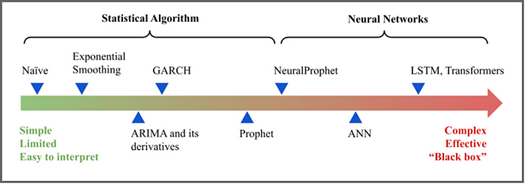# 时序相似性计算：从DTW到FastDTW

## DTW简介

DTW（Dynamic Time Warping）是一种用于比较时间序列之间相似性的算法。它可以有效地处理在时间轴上存在偏移、缩放和扭曲等变形的时间序列数据。DTW算法通过对两个时间序列进行动态规整，将它们按最优路径进行对齐，并计算它们之间的距离度量值。DTW算法被广泛应用于语音识别、图像识别、信号处理、生物信息学等领域。一个时间序列“扭曲”到一个示例如图所示：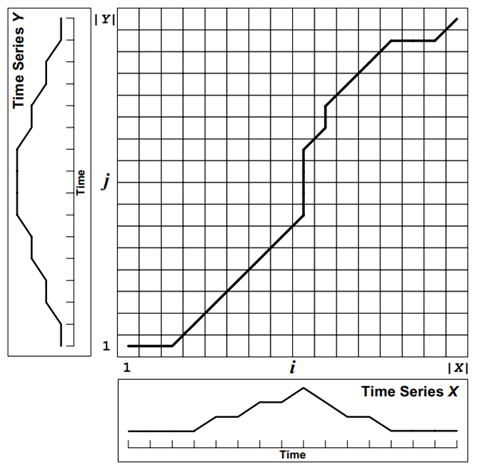### DTW基本原理

DTW通过把时间序列进行延伸和缩短，来计算两个时间序列性之间的相似性：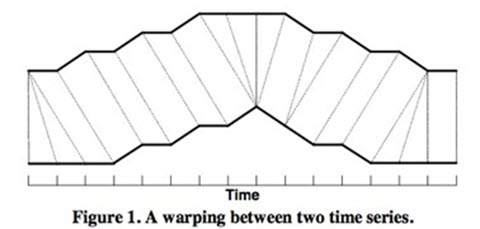$$X=x_1,x_2,…,x_N​$$

$$Y=y_1,y_2,…,y_M$$

• 初始化对齐路径的起点和终点为(1,1)和(N,M)，并定义一个二维矩阵D，其中$D(i, j)$表示序列$X_i$和$Y_j$之间的最小距离度量值。
• 递归计算矩阵D中每个元素的值，并选择路径上的最小值。公式如下：$D(i,j)=d(i,j)+min(D(i-1,j),D(i,j-1),D(i-1,j-1))$，其中d(i,j)表示序列$X_i$和$Y_j$之间的距离度量值，min()函数表示取三个参数的最小值。
• 最后，在矩阵D中沿最优路径从（1,1）到（N，M）遍历，即可得到最小距离度量值。

### DTW算法是如何工作的？

 Time Series_1 Series_2 1 1 1 2 7 2 3 4 8 4 8 5 5 2 5 6 9 1 7 6 9 8 5 4 9 2 6 10 0 5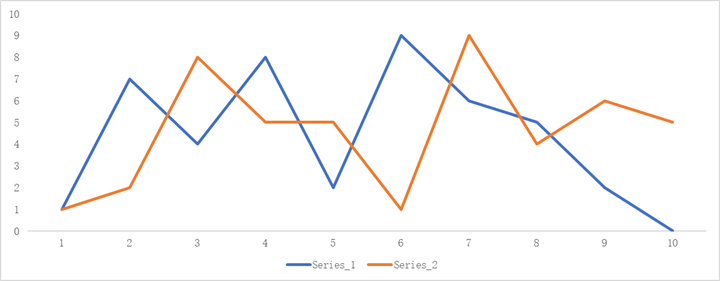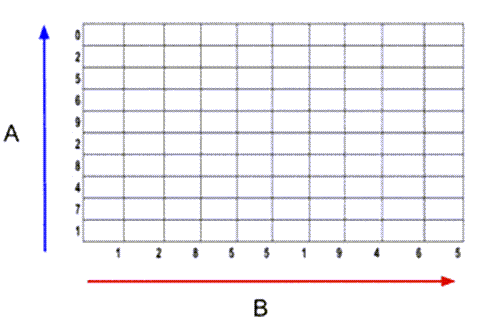$$M(i, j) = |A(i) – B(j)| + min (M(i-1,j-1), M(i, j-1), M(i-1,j))$$

• M是矩阵
• i是A系列的迭代器
• j是B系列的迭代器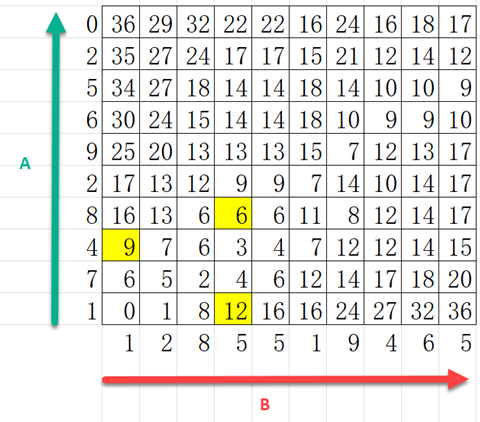• 对于6：|8 -5| + min(6, 6, 3) = 6
• 对于9：|4 -1| + min(6) = 9
• 对于12：|1 -5| + min(8) = 12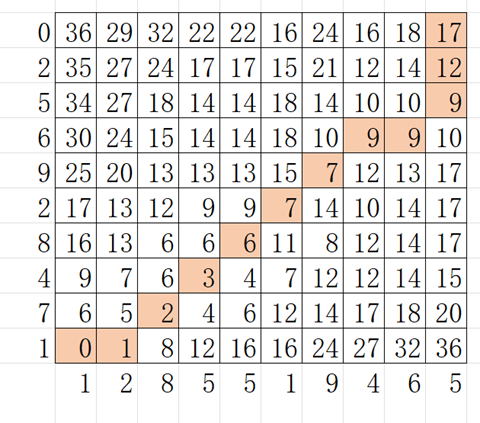d = [17, 12, 9, 9, 9, 7, 7, 6, 3, 2, 1, 0]

$$D = \frac{\sum_{i=1}^{k}d(i)}{\sum_{i=1}^{k}k}$$

D = (17 + 12 + 9 + 9 + 9 +7 + 7 + 6 + 3 + 2 +1 + 0)/12=6.8333

### DTW算法的优点和缺点

DTW算法具有以下优点：

• 能够有效地处理在时间轴上存在偏移、缩放和扭曲等变形的时间序列数据；
• 可以处理不同长度的时间序列，因此更加灵活；
• 算法简单易懂，容易实现。

• 对于长时间序列而言，计算复杂度很高，因此需要占用大量的计算资源；
• 算法在寻找最优路径时，可能会受到局部极小值的影响，导致结果不够准确；
• 算法对于噪声和异常值比较敏感，可能会带来一定的误差。

### DTW算法伪代码实现

int DTWDistance(s: array [1..n], t: array [1..m]) {
DTW := array [0..n, 0..m]

for i := 1 to n
DTW[i, 0] := infinity
for i := 1 to m
DTW[0, i] := infinity
DTW[0, 0] := 0

for i := 1 to n
for j := 1 to m
cost:= d(s[i], t[j])
DTW[i, j] := cost + minimum(DTW[i-1, j  ],    // insertion
DTW[i  , j-1],    // deletion
DTW[i-1, j-1])    // match

return DTW[n, m]
}


### DTW算法Python实现

import numpy as np
from dtw import dtw

x = np.array([2, 0, 1, 1, 2, 4, 2, 1, 2, 0])
y = np.array([1, 1, 2, 4, 2, 1, 4, 2])
manhattan_distance = lambda x, y: np.abs(x - y)

d, cost_matrix, acc_cost_matrix, path = dtw(x, y, dist=manhattan_distance)
print(d)


## FastDTW简介

DTW采用动态规划来计算两个时间序列之间的相似性，算法复杂度为$O(N^2)$。当两个时间序列都比较长时，DTW算法效率比较慢，不能满足需求，为此，有许多对DTW进行加速的算法：FastDTW,SparseDTW,LB_Keogh,LB_Improved等。在这里我们介绍FastDTW。

### FastDTW之前的优化方案

FastDTW之前，后来主要有两种近似计算DWT的算法，损失一定准确率的前提下减少时间和空间复杂度：

1、约束 – 限制在成本矩阵中评估的单元数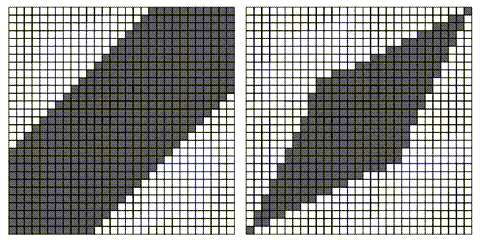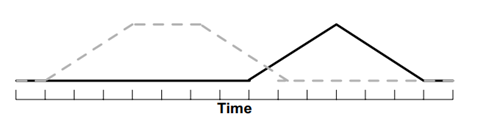2、抽象——对数据的简化表示执行 DTW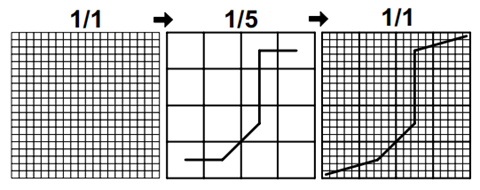FastDTW使用下面三种方法进行改进：

• 粗化——将时间序列缩小为更小的时间序列，以更少的数据点尽可能准确地表示相同的曲线。
• 投影——在较低分辨率下找到最小距离扭曲路径，并将其用作更高分辨率最小距离扭曲路径的初始猜测。
• 细化——通过局部调整扭曲路径来优化从较低分辨率投影的扭曲路径。

FastDTW中的分级使用更高分辨率计算，弥补了之前的Abstract的方法，原来只使用一次降低分辨率；FastDTW中的细化使用半径参数控制，弥补原来的Band的方法那种不灵活性，因为Band的方法需要依靠先验知识判断最优路径大概在那些位置；而半径参数只是作为分级粗化投影的一个补充。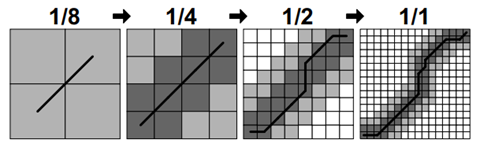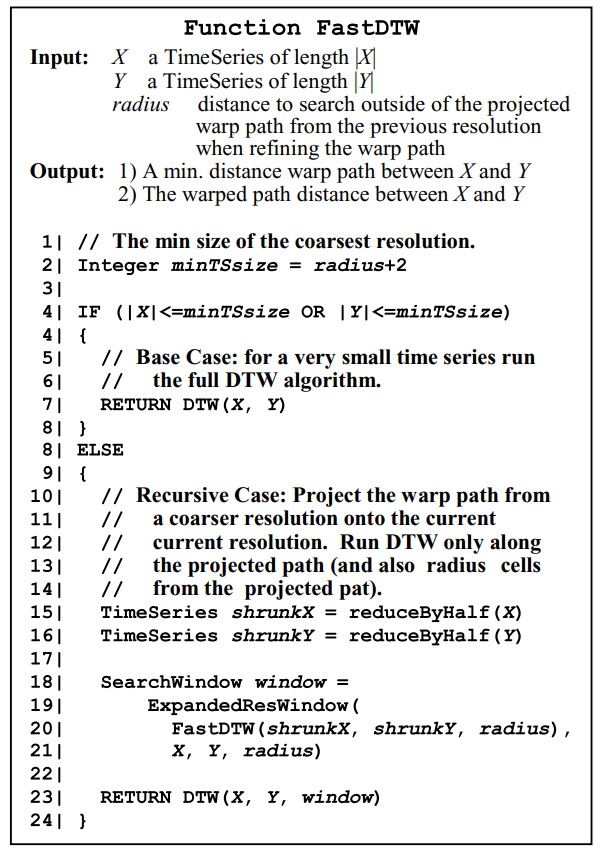### FastDTW的代码示例

import numpy as np
from scipy.spatial.distance import euclidean

from fastdtw import fastdtw

x = np.array([[1, 1], [2, 2], [3, 3], [4, 4], [5, 5]])
y = np.array([[2, 2], [3, 3], [4, 4]])
distance, path = fastdtw(x, y, dist=euclidean)
print(distance)


## DTW库比较

 Python包 平均执行时间 标准差 dtaidistance distance_fast 0.00011 0.00032 TSLearn 0.00021 0.00042 PyTS 0.00031 0.00046 DTW-Python 0.00144 0.00049 dtaidistance 0.03292 0.00171 FastDTW 0.05874 0.00246

### dtaidistance

dtaidistance是一个Python中的时间序列距离计算库，提供了多种距离度量方法和动态时间规整（DTW）算法的实现。它可以用于处理时间序列数据的相似性分析、聚类、分类等任务。

dtaidistance支持以下常见的时间序列距离度量方法：

• Euclidean：欧几里得距离，即两个时间序列之间点对点的欧式距离；
• Manhattan：曼哈顿距离，即两个时间序列之间点对点的曼哈顿距离；
• Chebyshev：切比雪夫距离，即两个时间序列之间点对点的切比雪夫距离；
• Minkowski：明可夫斯基距离，可用于在不同维度上加权考虑距离度量；
• Pearson：皮尔逊相关系数，可用于比较两条时间序列之间的线性关系。

dtaidistance提供了三种DTW算法的实现：

• 快速DTW（FastDTW）：通过使用特定的启发式策略和剪枝技术来减少计算时间，通常比标准DTW算法更快，并且可以在处理大型时间序列时提供更好的性能；
• 多尺度DTW（MultiscaleDTW）：将时间序列分解为多个尺度，并对每个尺度应用DTW算法，从而提高算法的鲁棒性和效率；
• 惩罚DTW（WarpingPathDTW）：在计算DTW距离时，额外惩罚路径偏移和扭曲，以尽可能使对齐路径保持连续和单调上升。

dtaidistance具有以下优点：

• 支持多种距离度量方法，可以根据不同的任务需求进行选择；
• 提供快速DTW、多尺度DTW和惩罚DTW等不同的DTW实现，可以根据数据大小和复杂性进行选择；
• 算法简单易懂，易于使用和扩展。

• 对于长时间序列而言，计算复杂度仍然较高，因此需要占用一定的计算资源；
• 算法对于噪声和异常值比较敏感，可能会带来一定的误差；
• 目前还不支持GPU加速，因此在处理大型数据时，可能会受到计算速度的限制。

from dtaidistance import dtw
import numpy as np

a = np.random.random(100)
b = np.random.random(200)

distance = dtw.distance(a, b)
print(f"DTW Distance: {distance}")

distance = dtw.distance_fast(a, b)
print(f"DTW Distance: {distance}")


### TSLearn

TSLearn是一个Python中的时间序列分析库，它提供了各种时间序列处理方法和机器学习算法的实现。TSLearn可以用于多种应用场景，例如时间序列分类、聚类、预测等任务。

TSLearn支持以下常见的时间序列处理方法和机器学习算法：

• 特征提取：TSLearn提供了多种特征提取方法，如峰值、波形长度、自相关等，可以将原始时间序列转化为一组有意义的特征向量；
• 相似性度量：TSLearn支持多种相似性度量方法，例如欧几里得距离、动态时间规整（DTW）距离、曼哈顿距离等，可以用于比较不同时间序列之间的相似性；
• 时间序列分类和聚类：TSLearn提供了多种分类和聚类算法，如KNN、SVM、随机森林、k-means等，可用于将时间序列进行分组或划分；
• 时间序列预测：TSLearn支持多种时间序列预测方法，如ARIMA模型、LSTM网络等，可用于预测未来时间序列的趋势和变化。

from tslearn.metrics import dtw as ts_dtw
import numpy as np

a = np.random.random((100, 2))
b = np.random.random((200, 2))

distance = ts_dtw(a, b)
print(f"DTW Distance: {distance}")


### PyTS DTW

PyTS DTW是一个Python中的时间序列距离计算库，它提供了多种基于动态时间规整（DTW）的距离度量方法。PyTS DTW可以用于处理时间序列数据的相似性分析、聚类、分类等任务。

PyTS DTW支持以下常见的基于DTW的时间序列距离度量方法：

• DTW：经典的动态时间规整算法，通过对两个时间序列进行动态规整，将它们按最优路径进行对齐，并计算它们之间的距离度量值；
• WDTW：权重动态时间规整算法，引入权重系数来平衡两条时间序列在DTW路径上不同位置的距离贡献；
• SINKHORN：Sinkhorn距离算法，通过迭代过程逼近两个时间序列之间的极小距离度量值；
• MSM：移动窗口匹配算法，将时间序列划分为多个子序列，并对每个子序列应用DTW算法，从而减少算法的计算复杂度。

PyTS DTW具有以下优点：

• 支持多种基于DTW的距离度量方法，可以根据不同的任务需求进行选择；
• 算法简单易懂，易于使用和扩展；
• 提供了可视化工具和示例代码，可帮助用户快速上手并理解算法原理。

• 对于长时间序列而言，计算复杂度仍然较高，可能需要占用大量的计算资源；
• 算法对于噪声和异常值比较敏感，需要对数据进行预处理和清洗；
• 部分算法的可调参数较多，需要进行参数调优才能达到最佳效果。

from pyts.metrics import dtw
import numpy as np

a = np.random.random((100, 2))
b = np.random.random((200, 2))

distance = dtw(a, b)

print(f"DTW Distance: {distance}")


### DTW-Python

DTW-Python是一个基于Python的动态时间规整（DTW）算法库，用于计算两个时间序列之间的距离度量值。它提供了多种DTW算法的实现和可视化工具，可用于处理时间序列数据的相似性分析、聚类、分类等任务。

DTW-Python提供了以下常见的DTW算法的实现：

• 经典DTW：通过对两个时间序列进行动态规整，将它们按最优路径进行对齐，并计算它们之间的距离度量值；
• 快速DTW：通过使用特定的启发式策略和剪枝技术来减少计算时间，通常比标准DTW算法更快，并且可以在处理大型时间序列时提供更好的性能；
• 条件DTW：在计算DTW距离时，引入一些先验信息来约束对齐路径，从而提高算法的准确性和鲁棒性；
• 级联DTW：将时间序列划分为多个子序列，并对每个子序列应用DTW算法，从而减少算法的计算复杂度。

DTW-Python具有以下优点：

• 支持多种DTW算法的实现，可以根据不同的任务需求进行选择；
• 提供可视化工具和示例代码，可帮助用户快速上手并理解算法原理；
• 代码简单易懂，易于使用和扩展。

• 对于长时间序列而言，计算复杂度仍然较高，可能需要占用大量的计算资源；
• 算法对于噪声和异常值比较敏感，需要对数据进行预处理和清洗；
• 目前还不支持GPU加速，因此在处理大型数据时，可能会受到计算速度的限制。

from dtw import dtw
import numpy as np

a = np.random.random((100, 2))
b = np.random.random((200, 2))

alignment = dtw(a, b)

print(f"DTW Distance: {alignment.distance}")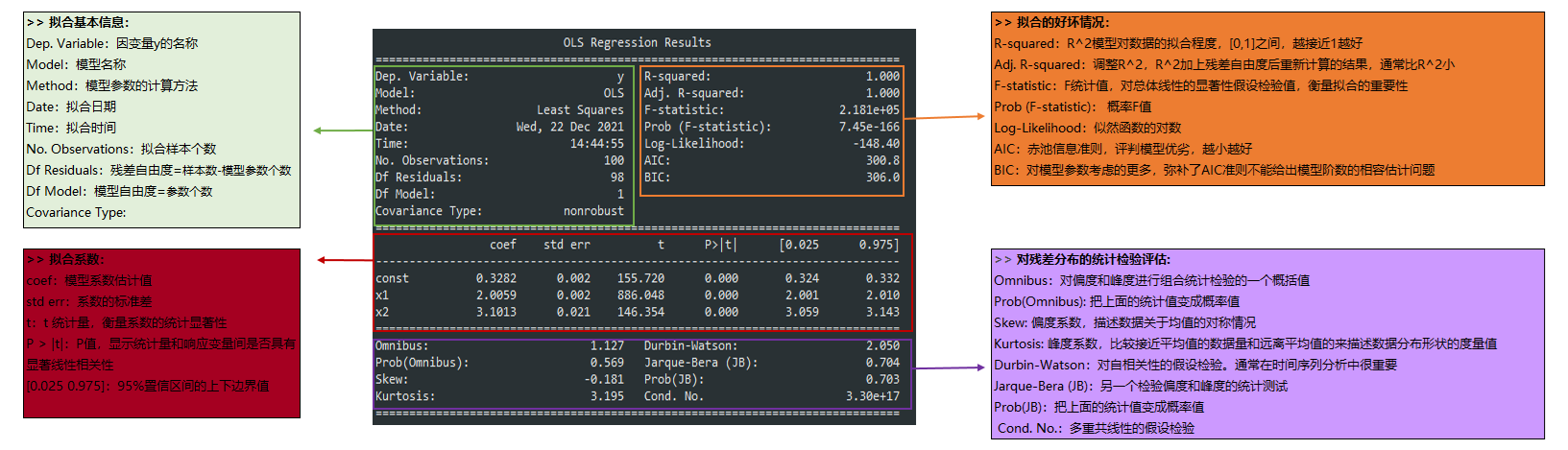##### Statsmodels 统计包之OLS回归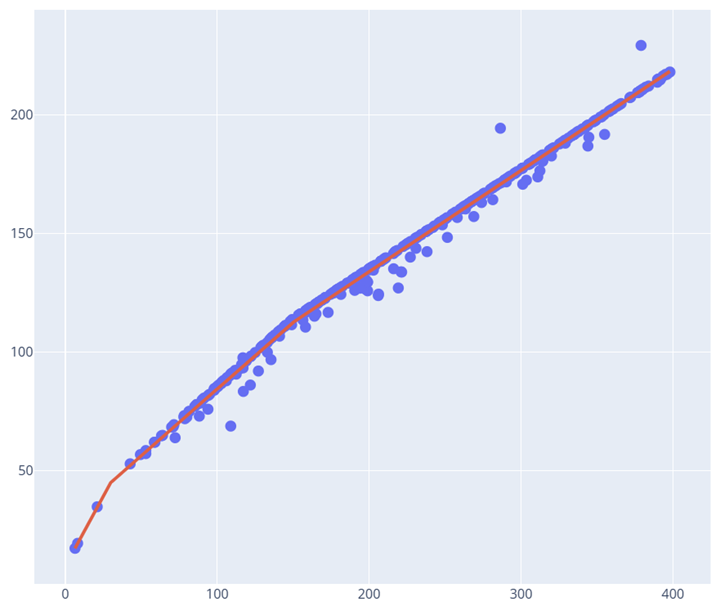##### 机器学习之分段线性回归